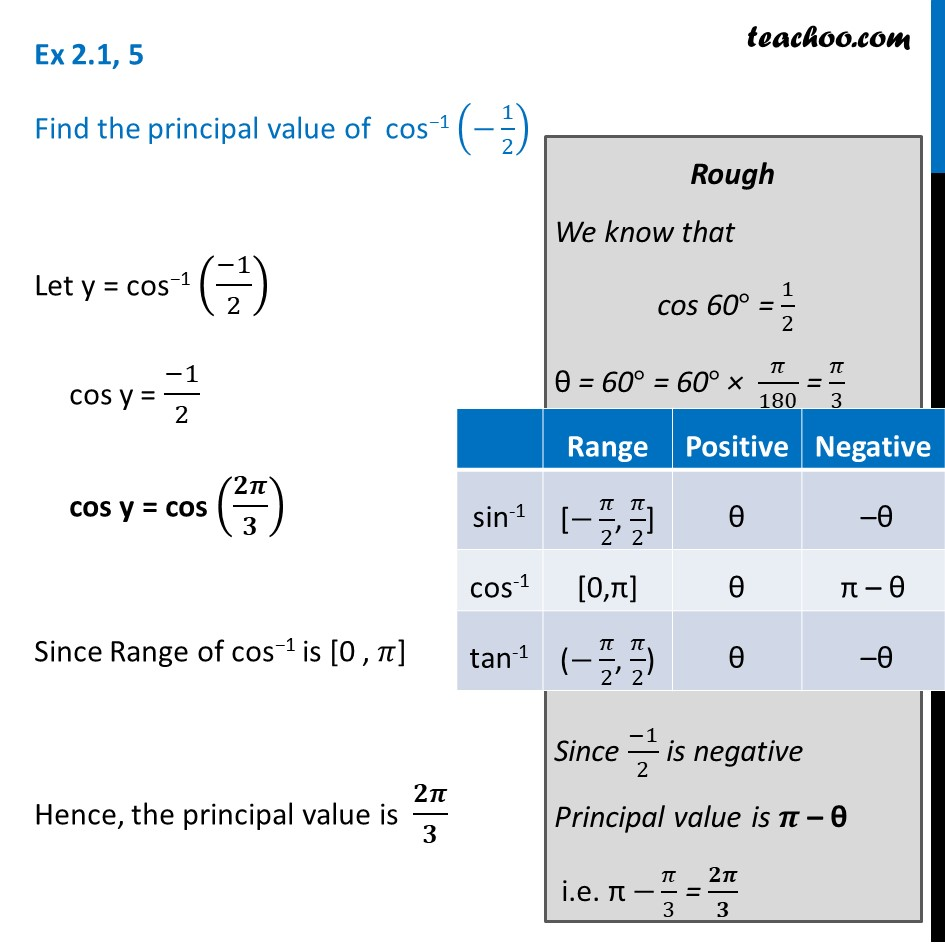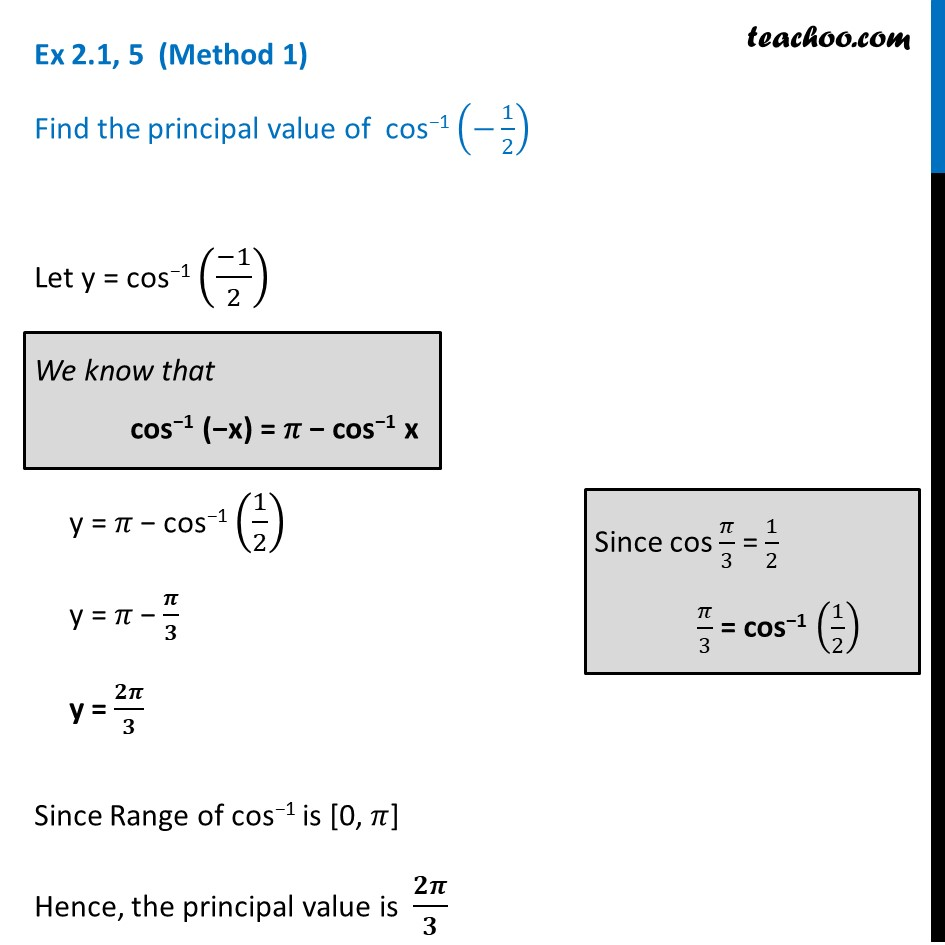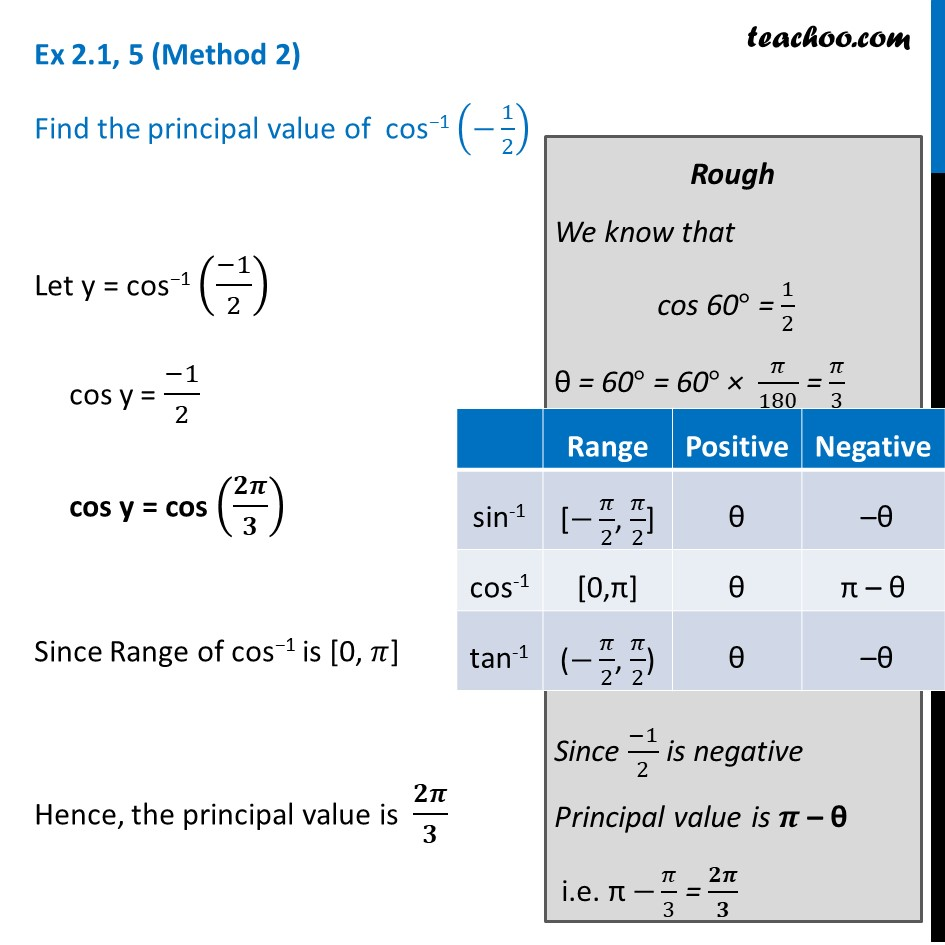Ex 2.1

Chapter 2 Class 12 Inverse Trigonometric Functions
Serial order wiseLearn in your speed, with individual attention - Teachoo Maths 1-on-1 Class

### Transcript

Ex 2.1, 5 Find the principal value of cos−1 (−1/2) Let y = cos−1 ((−1)/2) cos y = (−1)/2 cos y = cos (𝟐𝝅/𝟑) Since Range of cos−1 is [0 , 𝜋] Hence, the principal value is 𝟐𝝅/𝟑 Rough We know that cos 60° = 1/2 θ = 60° = 60° × 𝜋/180 = 𝜋/3 Since (−1)/2 is negative Principal value is 𝝅 – θ i.e. π −𝜋/3 = 𝟐𝝅/𝟑 Ex 2.1, 5 (Method 1) Find the principal value of cos−1 (−1/2) Let y = cos−1 ((−1)/2) y = 𝜋 − cos−1 (1/2) y = 𝜋 − 𝝅/𝟑 y = 𝟐𝝅/𝟑 Since Range of cos−1 is [0, 𝜋] Hence, the principal value is 𝟐𝝅/𝟑 We know that cos−1 (−x) = 𝜋 − cos−1 x Since cos 𝜋/3 = 1/2 𝜋/3 = cos−1 (1/2) Ex 2.1, 5 (Method 2) Find the principal value of cos−1 (−1/2) Let y = cos−1 ((−1)/2) cos y = (−1)/2 cos y = cos (𝟐𝝅/𝟑) Since Range of cos−1 is [0, 𝜋] Hence, the principal value is 𝟐𝝅/𝟑 Rough We know that cos 60° = 1/2 θ = 60° = 60° × 𝜋/180 = 𝜋/3 Since (−1)/2 is negative Principal value is 𝝅 – θ i.e. π −𝜋/3 = 𝟐𝝅/𝟑